# Marginal Independence and Conditional Independence Computer Science cpsc

• Slides: 24Marginal Independence and Conditional Independence Computer Science cpsc 322, Lecture 26 (Textbook Chpt 6. 1 -2) March, 19, 2010Lecture Overview • Recap with Example – Marginalization – Conditional Probability – Chain Rule • Bayes' Rule • Marginal Independence • Conditional Independence our most basic and robust form of knowledge about uncertain environments.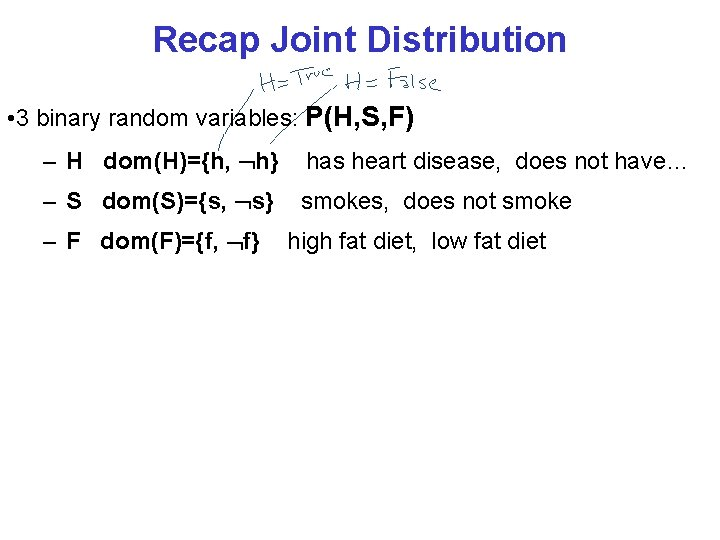Recap Joint Distribution • 3 binary random variables: P(H, S, F) – H dom(H)={h, h} has heart disease, does not have… – S dom(S)={s, s} smokes, does not smoke – F dom(F)={f, f} high fat diet, low fat dietRecap Joint Distribution • 3 binary random variables: P(H, S, F) – H dom(H)={h, h} has heart disease, does not have… – S dom(S)={s, s} smokes, does not smoke – F dom(F)={f, f} high fat diet, low fat diet f f s h h s s s . 015 . 007 . 005 . 003 . 21 . 51 . 07 . 18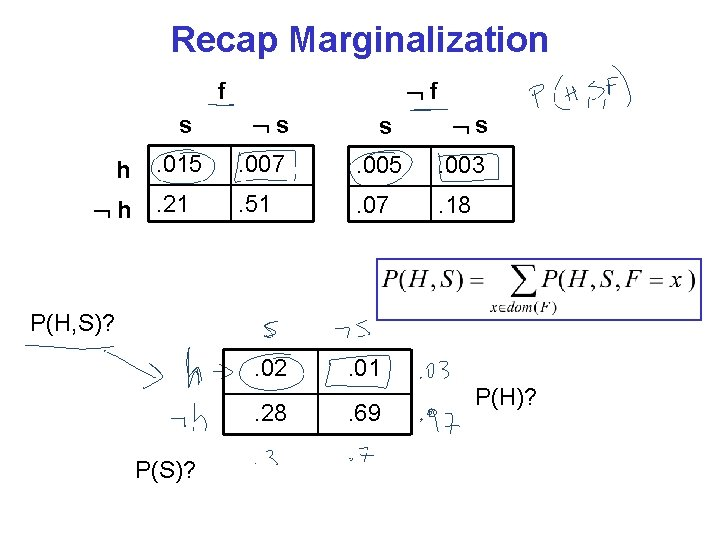Recap Marginalization f f s h h s s s . 015 . 007 . 005 . 003 . 21 . 51 . 07 . 18 P(H, S)? . 02. 28 P(S)? . 01. 69 P(H)?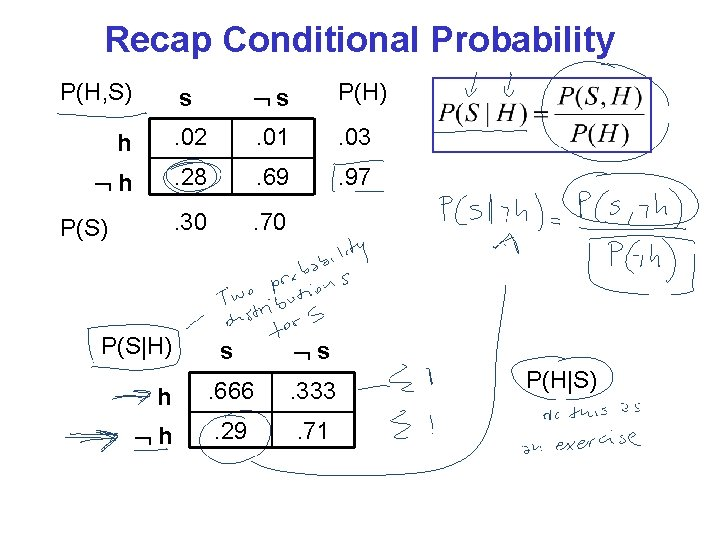Recap Conditional Probability P(H, S) s s P(H) h . 02 . 01 . 03 h . 28 . 69 . 97 . 30 . 70 P(S) P(S|H) s s h . 666 . 333 h . 29 . 71 P(H|S)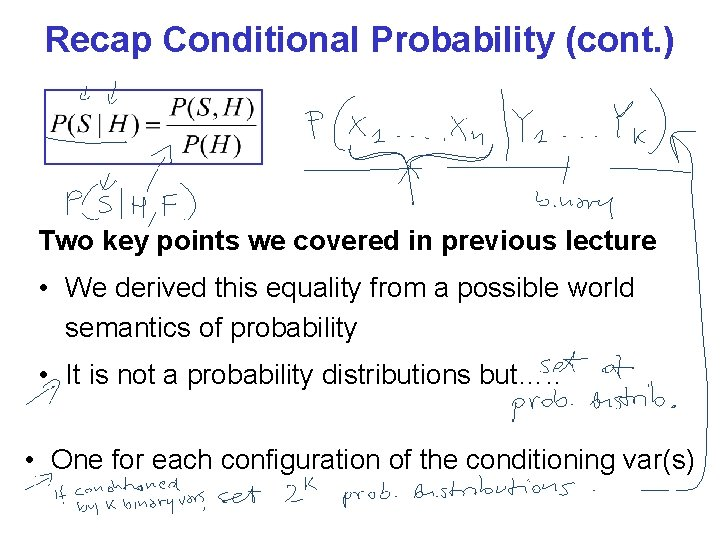Recap Conditional Probability (cont. ) Two key points we covered in previous lecture • We derived this equality from a possible world semantics of probability • It is not a probability distributions but…. . • One for each configuration of the conditioning var(s)Recap Chain Rule Bayes TheoremLecture Overview • Recap with Example and Bayes Theorem • Marginal Independence • Conditional IndependenceDo you always need to revise your beliefs? …… when your knowledge of Y’s value doesn’t affect your belief in the value of X DEF. Random variable X is marginal independent of random variable Y if, for all xi dom(X), yk dom(Y), P( X= xi | Y= yk) = P(X= xi )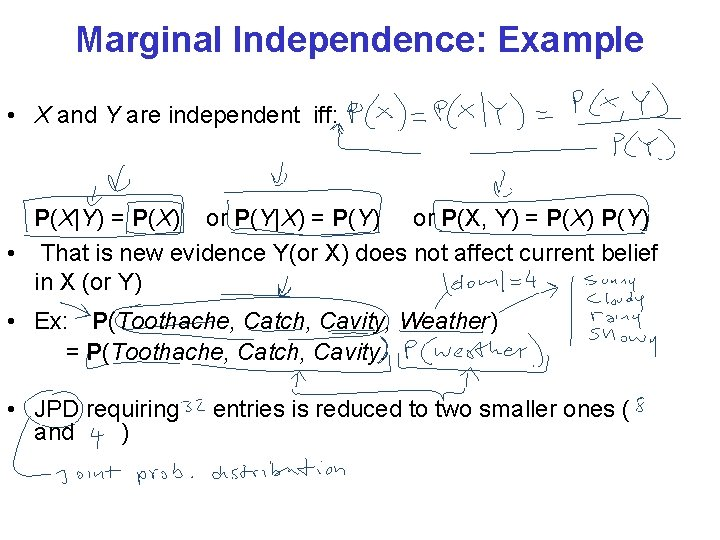Marginal Independence: Example • X and Y are independent iff: P(X|Y) = P(X) or P(Y|X) = P(Y) or P(X, Y) = P(X) P(Y) • That is new evidence Y(or X) does not affect current belief in X (or Y) • Ex: P(Toothache, Catch, Cavity, Weather) = P(Toothache, Catch, Cavity. • JPD requiring and ) entries is reduced to two smaller ones (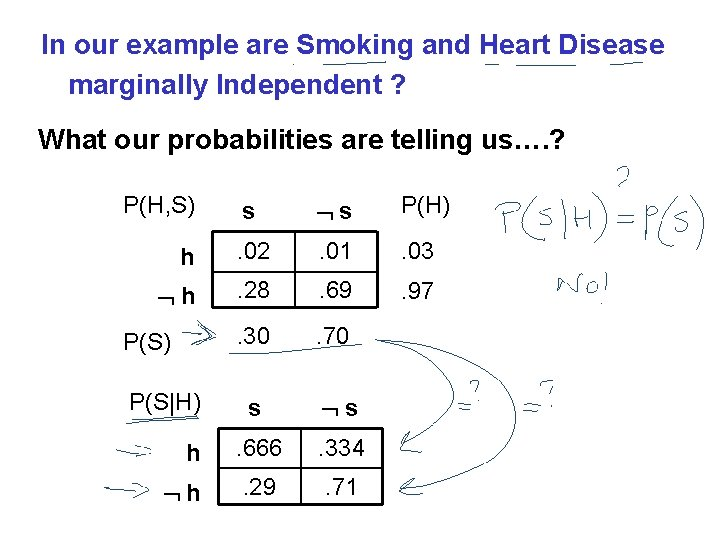In our example are Smoking and Heart Disease marginally Independent ? What our probabilities are telling us…. ? P(H, S) s s P(H) h . 02 . 01 . 03 h . 28 . 69 . 97 . 30 . 70 s s h . 666 . 334 h . 29 . 71 P(S) P(S|H)Lecture Overview • Recap with Example • Marginal Independence • Conditional Independence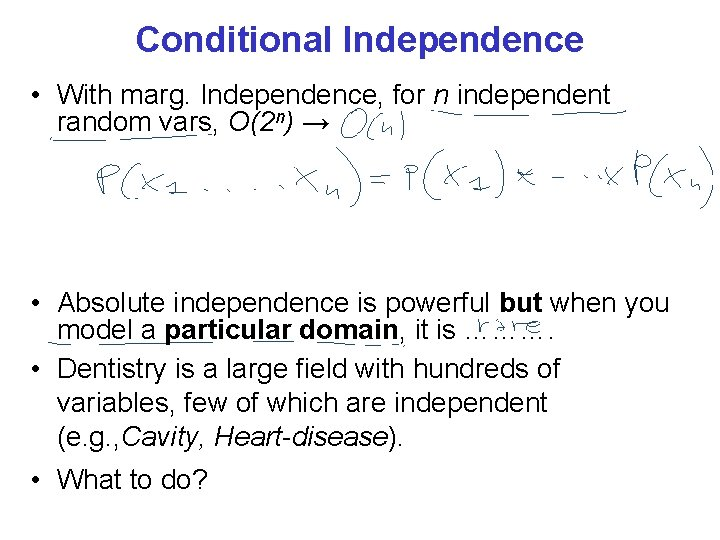Conditional Independence • With marg. Independence, for n independent random vars, O(2 n) → • Absolute independence is powerful but when you model a particular domain, it is ………. • Dentistry is a large field with hundreds of variables, few of which are independent (e. g. , Cavity, Heart-disease). • What to do?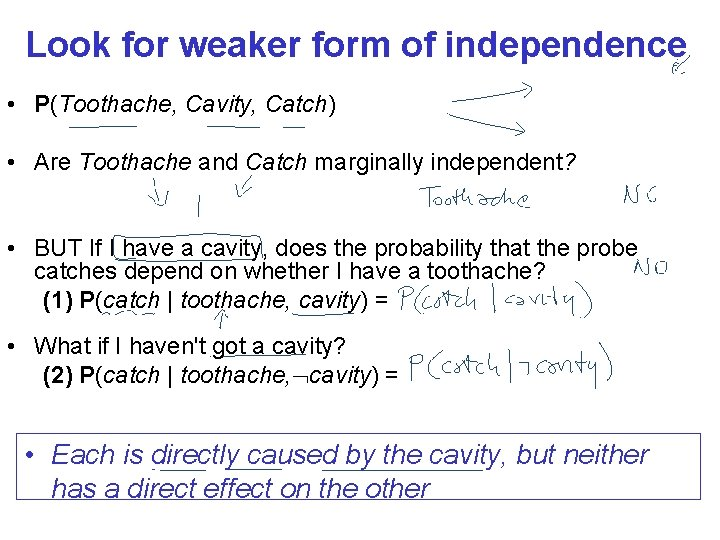Look for weaker form of independence • P(Toothache, Cavity, Catch) • Are Toothache and Catch marginally independent? • BUT If I have a cavity, does the probability that the probe catches depend on whether I have a toothache? (1) P(catch | toothache, cavity) = • What if I haven't got a cavity? (2) P(catch | toothache, cavity) = • Each is directly caused by the cavity, but neither has a direct effect on the other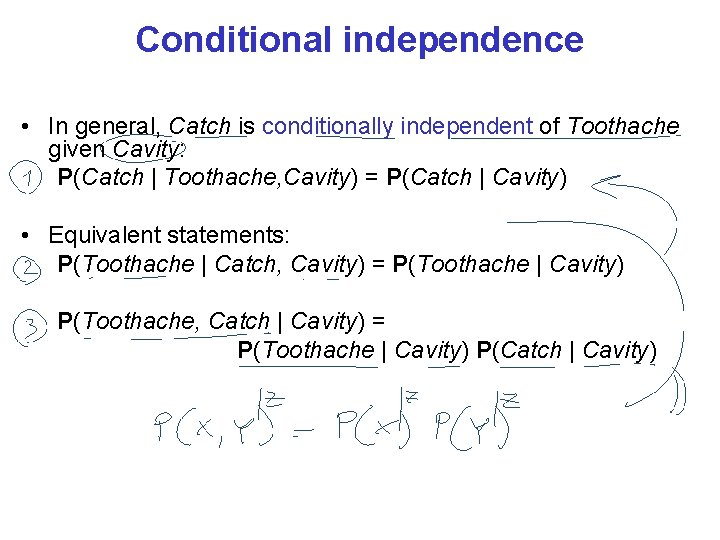Conditional independence • In general, Catch is conditionally independent of Toothache given Cavity: P(Catch | Toothache, Cavity) = P(Catch | Cavity) • Equivalent statements: P(Toothache | Catch, Cavity) = P(Toothache | Cavity) P(Toothache, Catch | Cavity) = P(Toothache | Cavity) P(Catch | Cavity)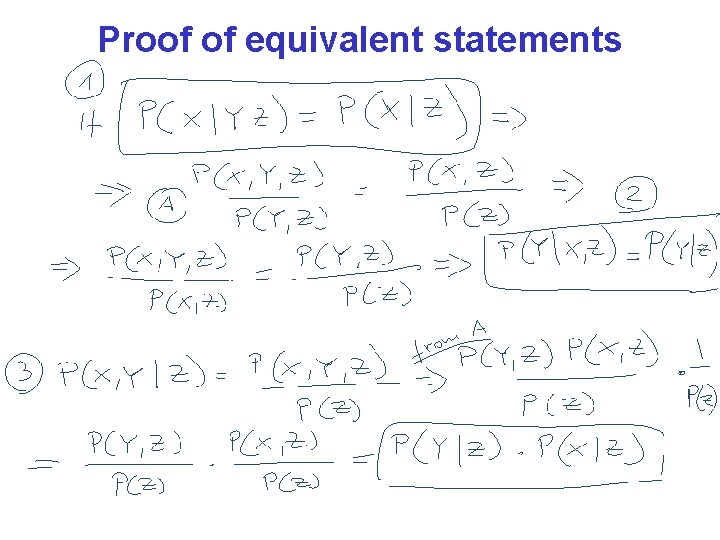Proof of equivalent statements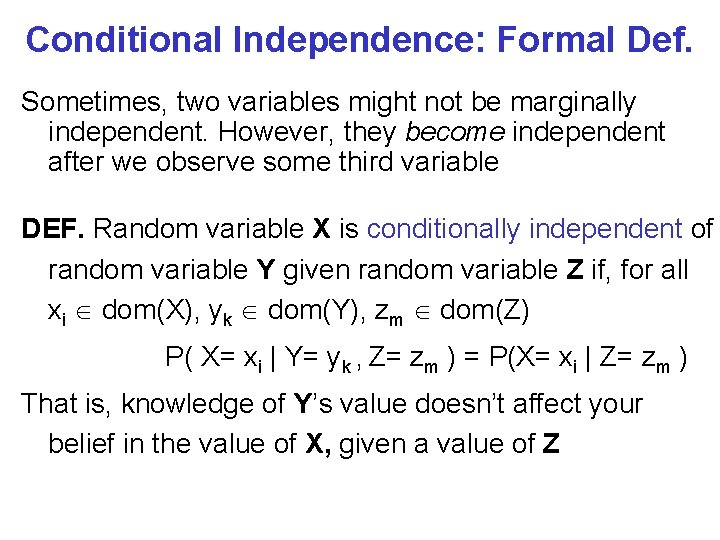Conditional Independence: Formal Def. Sometimes, two variables might not be marginally independent. However, they become independent after we observe some third variable DEF. Random variable X is conditionally independent of random variable Y given random variable Z if, for all xi dom(X), yk dom(Y), zm dom(Z) P( X= xi | Y= yk , Z= zm ) = P(X= xi | Z= zm ) That is, knowledge of Y’s value doesn’t affect your belief in the value of X, given a value of Z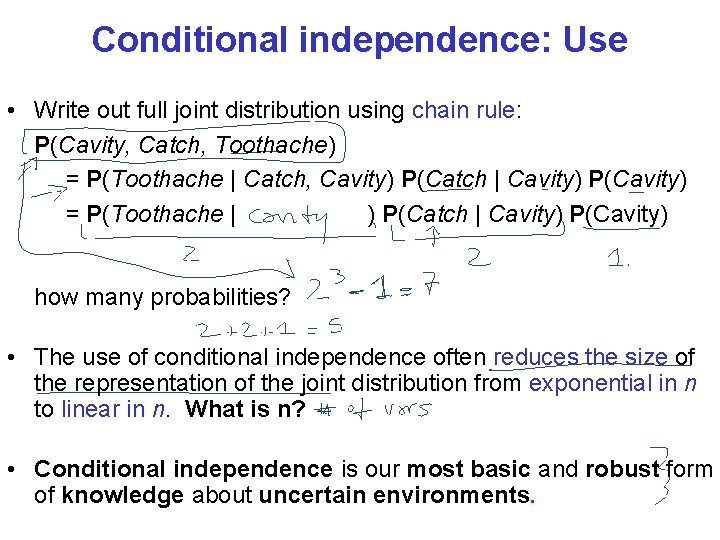Conditional independence: Use • Write out full joint distribution using chain rule: P(Cavity, Catch, Toothache) = P(Toothache | Catch, Cavity) P(Catch | Cavity) P(Cavity) = P(Toothache | ) P(Catch | Cavity) P(Cavity) how many probabilities? • The use of conditional independence often reduces the size of the representation of the joint distribution from exponential in n to linear in n. What is n? • Conditional independence is our most basic and robust form of knowledge about uncertain environments.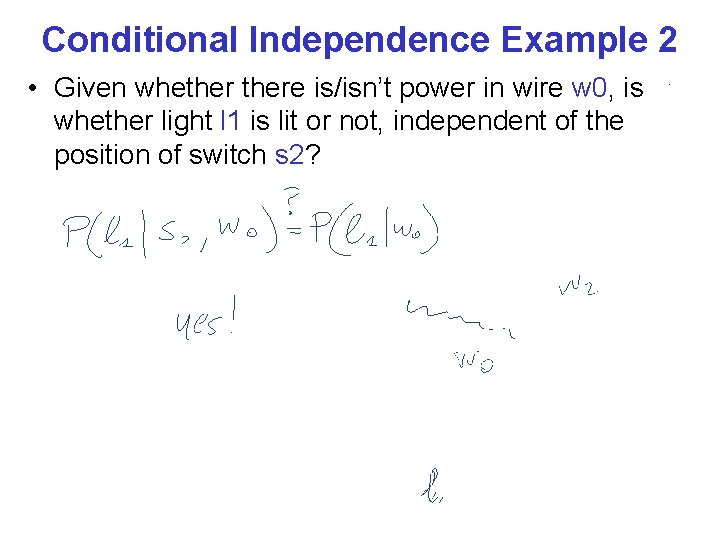Conditional Independence Example 2 • Given whethere is/isn’t power in wire w 0, is whether light l 1 is lit or not, independent of the position of switch s 2?Conditional Independence Example 3 • Is every other variable in the system independent of whether light l 1 is lit, given whethere is power in wire w 0 ?Learning Goals for today’s class • You can: • Derive the Bayes Rule • Define and use Marginal Independence • Define and use Conditional Independence CPSC 322, Lecture 4 Slide 22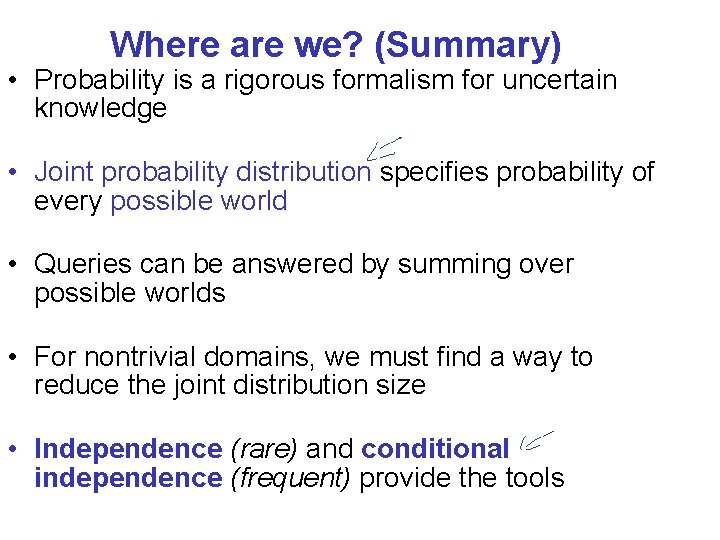Where are we? (Summary) • Probability is a rigorous formalism for uncertain knowledge • Joint probability distribution specifies probability of every possible world • Queries can be answered by summing over possible worlds • For nontrivial domains, we must find a way to reduce the joint distribution size • Independence (rare) and conditional independence (frequent) provide the toolsNext Class • Bayesian Networks (Chpt 6. 3)